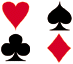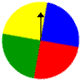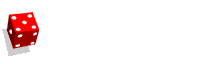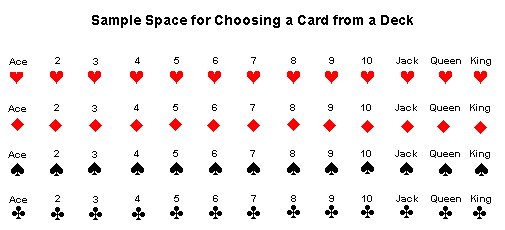# Understanding and How To Find Sample Space# What is Sample Space and How to Find Sample Space

Definition: The sample space of an experiment is the set of all possible outcomes of that experiment.Experiment 1: What is the probability of each outcome when a dime is tossed?

Outcomes: The outcomes of this experiment are head and tail.

Probabilities:

 P(head) = 1 2 P(tail) = 1 2

The sample space of Experiment 1 is:   {head, tail}

Experiment 2: A spinner has 4 equal sectors colored yellow, blue, green and red. What is the probability of landing on each color after spinning this spinner?Sample Space: {yellow, blue, green, red}

Probabilities:

 P(yellow) = 1 4 P(blue) = 1 4 P(green) = 1 4 P(red) = 1 4

Experiment 3: What is the probability of each outcome when a single 6-sided die is rolled?Sample Space: {1, 2, 3, 4, 5, 6}

Probabilities:

 P(1) = 1 6 P(2) = 1 6 P(3) = 1 6 P(4) = 1 6 P(5) = 1 6 P(6) = 1 6Experiment 4: A glass jar contains 1 red, 3 green, 2 blue and 4 yellow marbles. If a single marble is chosen at random from the jar, what is the probability of each outcome?

Sample Space: {red, green, blue, yellow}

Probabilities:

 P(red) = 1 10 P(green) = 3 10 P(blue) = 2 = 1 10 5 P(yellow) = 4 = 2 10 5

Summary: The sample space of an experiment is the set of all possible outcomes for that experiment. You may have noticed that for each of the experiments above, the sum of the probabilities of each outcome is 1. This is no coincidence. The sum of the probabilities of the distinct outcomes within a sample space is 1.

The sample space for choosing a single card at random from a deck of 52 playing cards is shown below. There are 52 possible outcomes in this sample space.The probability of each outcome of this experiment is:

 P(card) = 1 52

The sum of the probabilities of the distinct outcomes within this sample space is:

 52 = 1 52

### Exercises

Directions: Read each question below. Select your answer by clicking on its button. Feedback to your answer is provided in the RESULTS BOX. If you make a mistake, choose a different button.

 1. What is the sample space for choosing an odd number from 1 to 11 at random? 1, 2, 3, 4, 5, 6, 7, 8, 9, 10, 11{1, 2, 3, 4, 5, 6, 7, 8, 9, 10, 11}{1, 3, 5, 7, 9 11}None of the above. RESULTS BOX:
 2. What is the sample space for choosing a prime number less than 15 at random? {2, 3, 5, 7, 11, 13, 15}{2, 3, 5, 7, 11, 13}{2, 3, 5, 7, 9, 11, 13}All of the above. RESULTS BOX:
 3. What is the sample space for choosing 1 jelly bean at random from a jar containing 5 red, 7 blue and 2 green jelly beans? {5, 7, 2}{5 red, 7 blue, 2 green}{red, blue, green}None of the above. RESULTS BOX:
 4. What is the sample space for choosing 1 letter at random from 5 vowels? {a, e, i, o, u}{v, o, w, e, l}{1, 2, 3, 4, 5}None of the above. RESULTS BOX:
 5. What is the sample space for choosing 1 letter at random from the word DIVIDE? {d, i, v, i, d, e}{1, 2, 3, 4, 5, 6}{d, i, v, e}None of the above. RESULTS BOX: